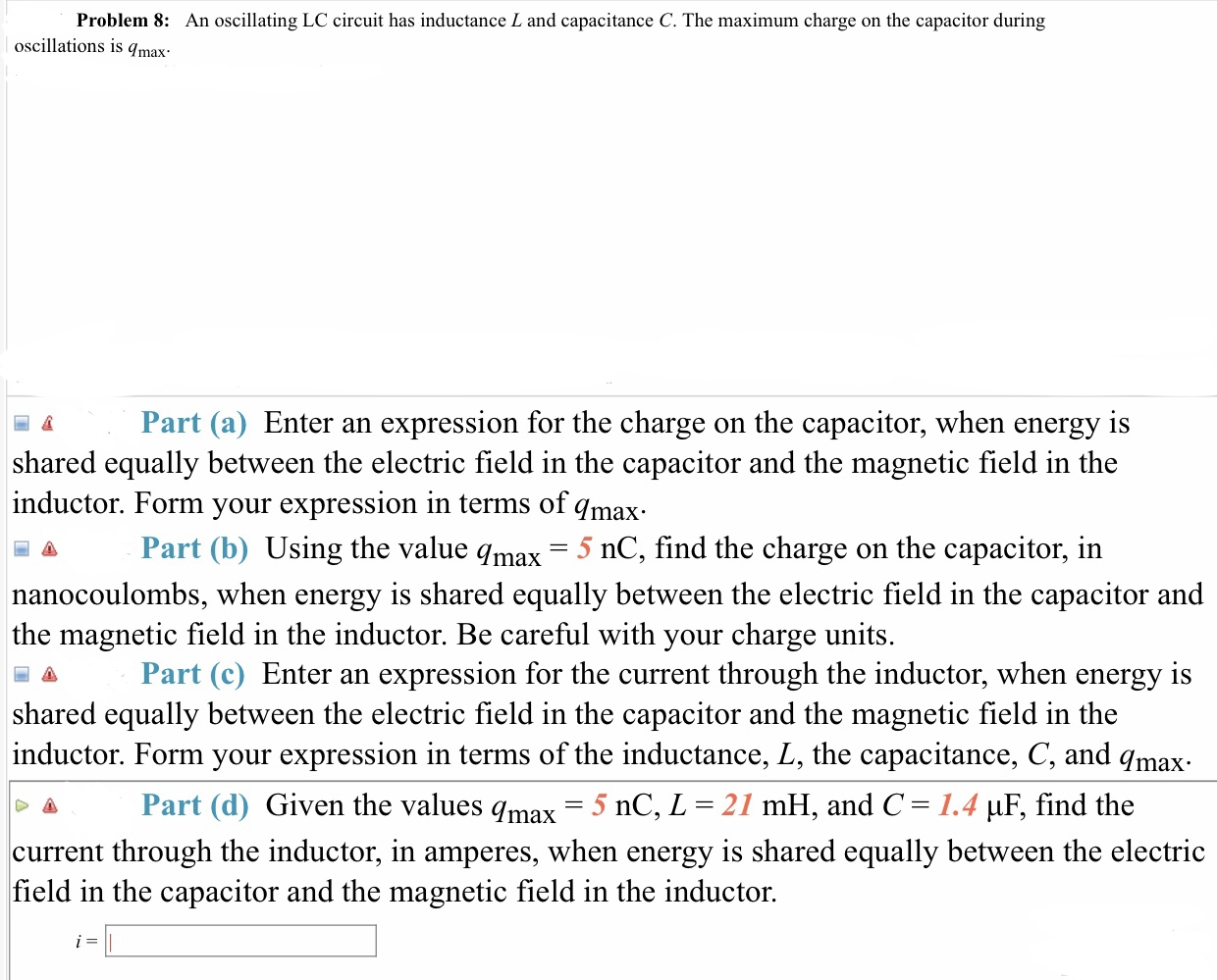# Problem 8:An oscillating LC circuit has inductance L and capacitance C. The maximum charge on the capacitor duringoscillations is qmaa Part (a) Enter an expression for the charge on the capacitor, when energy isshared equally between the electric field in the capacitor and the magnetic field in theinductor. Form your expression in terms of qmaxPart (b) Using the value qmax = 5 nC, find the charge on the capacitor, innanocoulombs, when energy is shared equally between the electric field in the capacitor andthe magnetic field in the inductor. Be careful with your charge units.Part (c) Enter an expression for the current through the inductor, when energy isshared equally between the electric field in the capacitor and the magnetic field in theinductor. Form your expression in terms of the inductance, L, the capacitance, C, and qmaxΔ Part (d) Given the values qmax-5 nC, L 21 mH, and C 1.4 μF, find thecurrent through the inductor, in amperes, when energy is shared equally between the electricfield in the capacitor and the magnetic field in the inductor.

Question
1890 viewshelp_outlineImage TranscriptioncloseProblem 8: An oscillating LC circuit has inductance L and capacitance C. The maximum charge on the capacitor during oscillations is qma a Part (a) Enter an expression for the charge on the capacitor, when energy is shared equally between the electric field in the capacitor and the magnetic field in the inductor. Form your expression in terms of qmax Part (b) Using the value qmax = 5 nC, find the charge on the capacitor, in nanocoulombs, when energy is shared equally between the electric field in the capacitor and the magnetic field in the inductor. Be careful with your charge units. Part (c) Enter an expression for the current through the inductor, when energy is shared equally between the electric field in the capacitor and the magnetic field in the inductor. Form your expression in terms of the inductance, L, the capacitance, C, and qmax Δ Part (d) Given the values qmax-5 nC, L 21 mH, and C 1.4 μF, find the current through the inductor, in amperes, when energy is shared equally between the electric field in the capacitor and the magnetic field in the inductor. fullscreen
check_circle

Step 1

Part (a)

The expression for maximum energy stored in the capacitor is,

Step 2

If the energy stored in capacitor is half of the maximum energy means then, the expression is given by,

Step 3

Part (b)

The expression for energy stored in the charged capacitor, if the energy i...

### Want to see the full answer?

See Solution

#### Want to see this answer and more?

Solutions are written by subject experts who are available 24/7. Questions are typically answered within 1 hour.*

See Solution
*Response times may vary by subject and question.
Tagged in

### Physics